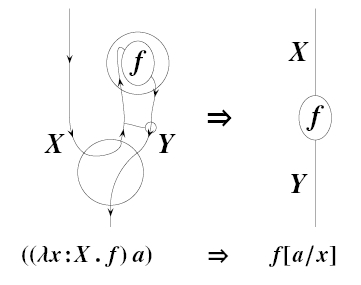## Computation and the Periodic TableIn physics, Feynman diagrams are used to reason about quantum processes. Similar diagrams can also be used to reason about logic, where they represent proofs, and computation, where they represent programs. With the rise of topological quantum field theory and quantum computation, it became clear that diagrammatic reasoning takes advantage of an extensive network of interlocking analogies between physics, topology, logic and computation. These analogies can be made precise using the formalism of symmetric monoidal closed categories. But symmetric monoidal categories are just the n = 1, k = 3 entry of a hypothesized "periodic table" of k-tuply monoidal n-categories. This raises the question of how these analogies extend. An important clue comes from the way symmetric monoidal closed 2-categories describe rewrite rules in the lambda calculus and multiplicative intuitionistic linear logic. This talk is based on work in progress with Paul-André Melličs and Mike Stay.

Click on this to see the transparencies of the talk:

For more details try the following papers:
Also try my seminar notes from Fall 2006 and Winter 2007.